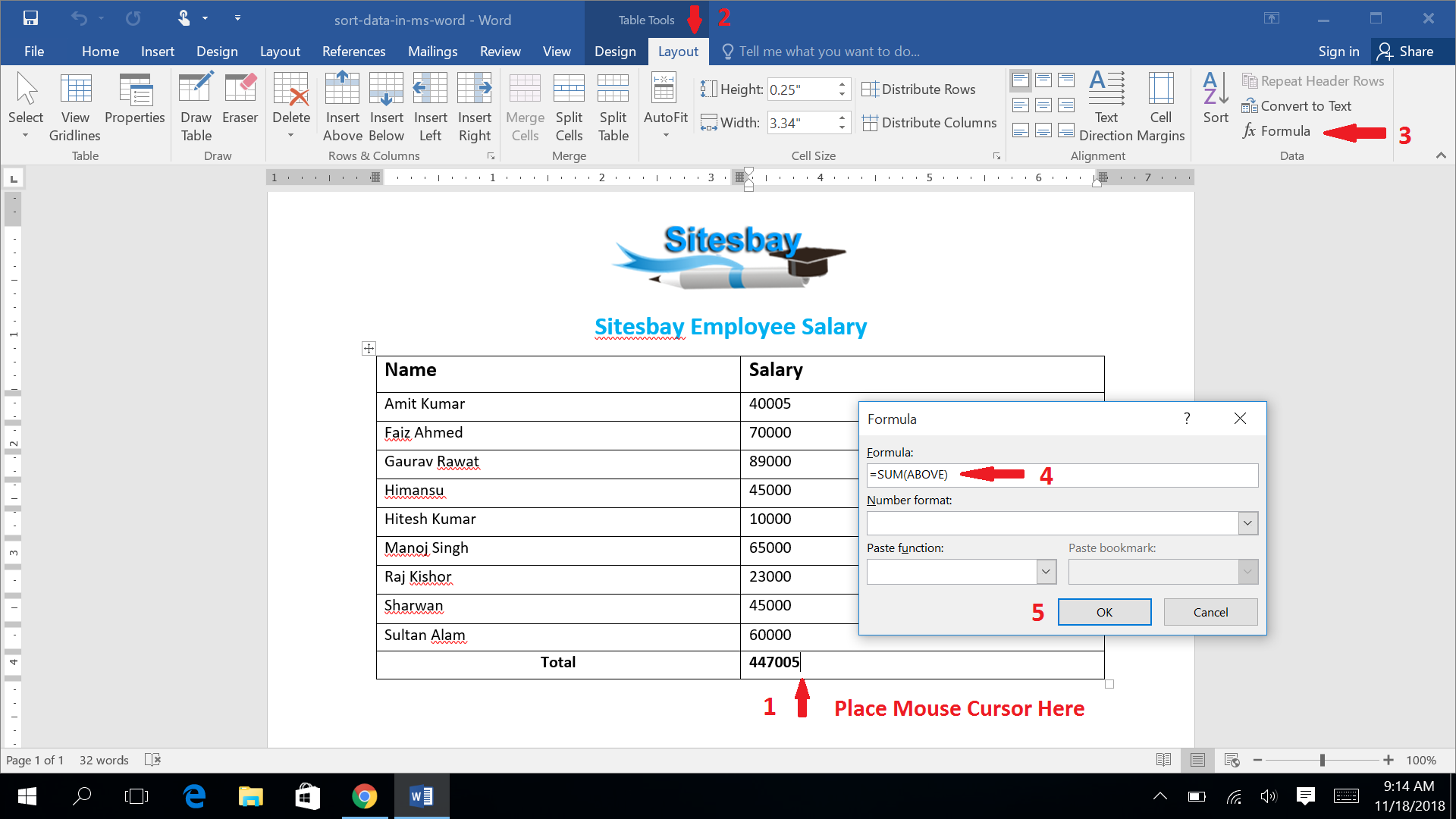# Add Sum Formula in MS-Word

## How to Add Sum Formula in MS-Word

Yes, this is right you can add sum formul in ms word. For add sum formula in word your data must be in table. To add sum formula in MS Word, Place your cursor at last row where your want sum of all above data. Steps for add sum formula in word are;

• Fill your data in table
• Place mouse curso at last row where you want sum of all above data.
• Visit Layout Tab
• Choose Formula command
• Job Done